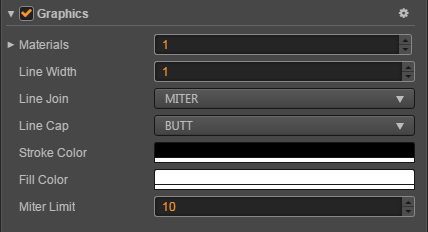# Graphics Component Reference

The Graphics component provides a series of drawing functions that reference the Web canvas's drawing APIs.Select a node in the Node Tree panel, and then click the Add Component button below the Properties panel and select Graphics from Renderer Component. Then you can add the Graphics component to the node.

## Graphic Properties

property Function Explanation
lineCap lineCap determines how the end points of every line are drawn
lineJoin lineJoin determines how two connecting segments (of lines, arcs or curves) with non-zero lengths in a shape are joined together
lineWidth Current line width
miterLimit Sets the miter limit ratio
strokeColor stroke color
fillColor fill color

## Graphics API

### Path

Function Function Explanation
moveTo (x, y) Move the render cursor to a specified point in the canvas without creating lines.
lineTo (x, y) Adds a straight line to the path
bezierCurveTo (c1x, c1y, c2x, c2y, x, y) Adds a cubic Bézier curve to the path
quadraticCurveTo (cx, cy, x, y) Adds a quadratic Bézier curve to the path
arc (cx, cy, r, a0, a1, counterclockwise) Adds an arc to the path which is centered at (cx, cy) position with radius r starting at startAngle and ending at endAngle going in the given direction by counterclockwise (defaulting to false).
ellipse (cx, cy, rx, ry) Adds an ellipse to the path
circle (cx, cy, r) Adds a circle to the path
rect (x, y, w, h) Adds a rectangle to the path
close () Adds an round corner rectangle to the path
stroke () Draws a filled rectangle
fill () Erasing any previously drawn content
clear () Causes the point of the pen to move back to the start of the current path. It tries to add a straight line from the current point to the start